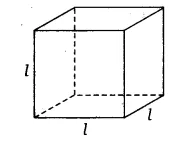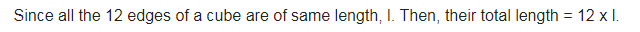# A cube is a three-dimensional figure as shown in figure

A cube is a three-dimensional figure as shown in figure. It has six faces and all of them are identical squares. The length of an edge of the cube is given by l. Find the formula for the total length of the edges of a cube.